This assignment is separate from your project. Include your answers in this document in the sections below the rubric.

Answer the questions with the three sampling/data examples.

library(tidyverse)
## -- Attaching packages --------------------------------------------------------------------------- tidyverse 1.2.1 --
## v ggplot2 3.1.0     v purrr   0.3.0
## v tibble  2.0.1     v dplyr   0.7.8
## v tidyr   0.8.2     v stringr 1.3.1
## v readr   1.3.1     v forcats 0.3.0
## -- Conflicts ------------------------------------------------------------------------------ tidyverse_conflicts() --
## x dplyr::filter() masks stats::filter()
## x dplyr::lag()    masks stats::lag()

# Lego example 1, hand-drawn sample

## Draw samples, estimate the mean “cells” of 50 Lego assemblages

A “cell” is defined as a one-unit high square that includes a single lego circle. Some assemblages have a 1/5-high base; ignore this, it is only for structure.

Procedure:

1. Select a “representative or random sample” of 5 assemblages out of the bin.
2. Count the number of cells for each assemblage.
3. Calculate the sample mean of the 5 (to estimate population mean of the 50); you can use this R code by replacing the numbers: mean(c(1, 2, 3, 4, 5)).
4. Write your mean cell-count estimate on a portable white board in big numbers (3 decimal places) and lay it on your table (so other tables don’t see).
5. When all tables are done, hang up your board so everyone can see each table’s cell-count estimate.
6. Record all the estimates in the R code chunk below and plot the estimate of the sampling distribution of the mean cell count based on $$n = 5$$.
# enter list of cells means here
sam.lego <-
read_csv("https://statacumen.com/teach/S4R/worksheet/S4R_WS_16_Sampling_LegoAssemblages_Data_SampleMeans.csv")
## Parsed with column specification:
## cols(
##   mean.cells = col_double()
## )
# we'll fill this in after data is collected
true.mean.cells = 11.86

summary(sam.lego$mean.cells) ## Min. 1st Qu. Median Mean 3rd Qu. Max. ## 2.60 3.75 11.50 19.48 28.35 68.00 # plot data and create table library(ggplot2) p <- ggplot(sam.lego, aes(x = mean.cells)) p <- p + geom_histogram(binwidth = 5) p <- p + geom_rug(alpha = 1/2) # est mean cells p <- p + geom_vline(aes(xintercept = mean(sam.lego$mean.cells))
, colour = "blue", size = 1)
# true mean cells
p <- p + geom_vline(aes(xintercept = true.mean.cells)
, colour = "red", linetype = "dotted", size = 1)
p <- p + labs(title = "Sampling distribution of mean cells of 50 assemblages\nn=5, red dotted = true, blue = est")
print(p)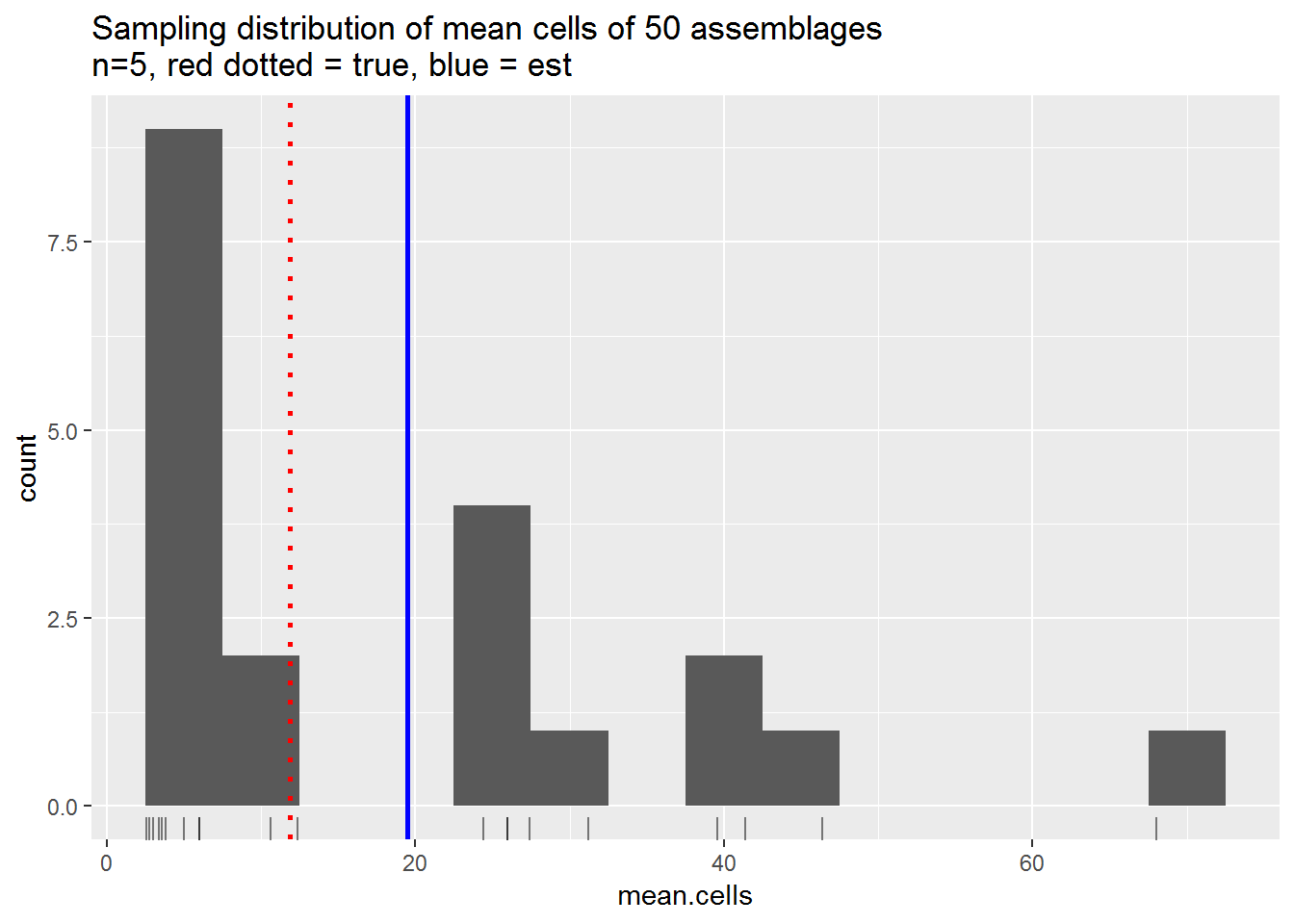## Questions to answer

1. (1 p) Adjust the histogram binwidth= to provide an informative representation of the distribution.

2. (1 p) Describe the sampling distribution: mention the center, spread, and any outliers in the plot.

3. (2 p) Are the estimates larger or smaller than the actual mean cells of the assemblages, and what might cause this bias?

# Lego example 2, simple random sample

## Draw samples, estimate the mean “cells” of 50 Lego assemblages

Procedure:

1. Select a “simple random sample” of 5 assemblages by drawing 5 random numbers (sample(x = 1:50, size = 5, replace = FALSE)) and counting the cells from the Lego assemblages worksheet, S4R_WS_16_Sampling_LegoAssemblages.pdf.
2. Count the number of cells for each assemblage.
3. Calculate the sample mean of the 5 (to estimate population mean of the 50); you can use this R code by replacing the numbers: mean(c(1, 2, 3, 4, 5)).
4. Write your mean cell-count estimate on a portable white board in big numbers (3 decimal places) and lay it on your table (so other tables don’t see).
5. When all tables are done, hang up your board so everyone can see each table’s cell-count estimate.
6. Record all the estimates in the R code chunk below and plot the estimate of the sampling distribution of the mean cell count based on $$n = 5$$.
# enter list of cells means here
sam.lego <-
read_csv("https://statacumen.com/teach/S4R/worksheet/S4R_WS_16_Sampling_LegoAssemblages_Data_SampleMeansSRS.csv")
## Parsed with column specification:
## cols(
##   mean.cells = col_double()
## )
# we'll fill this in after data is collected
true.mean.cells = 11.86

summary(sam.lego$mean.cells) ## Min. 1st Qu. Median Mean 3rd Qu. Max. ## 2.00 2.95 11.40 11.59 16.80 30.60 # plot data and create table library(ggplot2) p <- ggplot(sam.lego, aes(x = mean.cells)) p <- p + geom_histogram(binwidth = 5) p <- p + geom_rug(alpha = 1/2) # est mean cells p <- p + geom_vline(aes(xintercept = mean(sam.lego$mean.cells))
, colour = "blue", size = 1)
# true mean cells
p <- p + geom_vline(aes(xintercept = true.mean.cells)
, colour = "red", linetype = "dotted", size = 1)
p <- p + labs(title = "Sampling distribution of mean cells of 50 assemblages\nn=5, red dotted = true, blue = est")
print(p)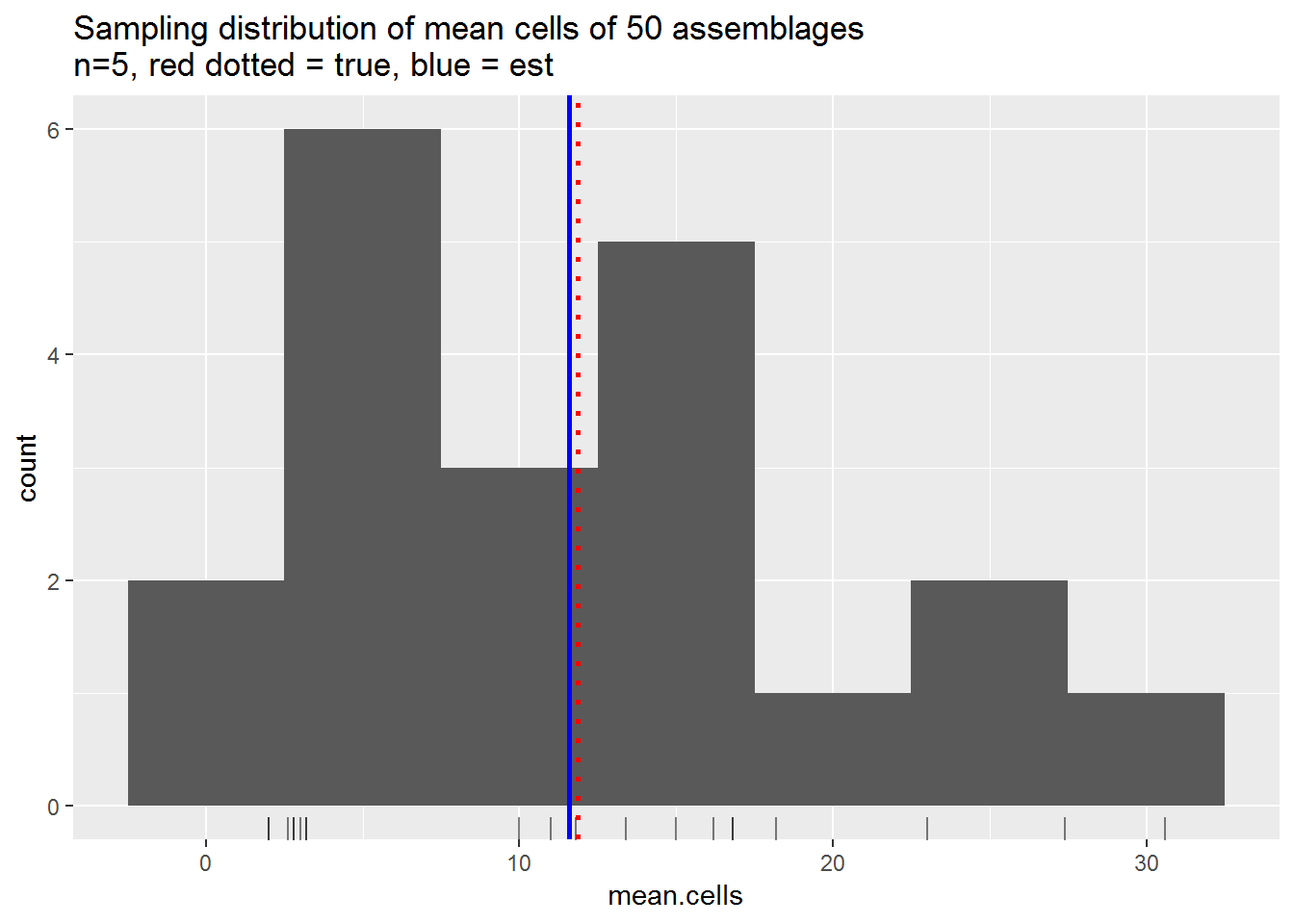## Questions to answer

1. (0 p) Adjust the histogram binwidth= to provide an informative representation of the distribution.

2. (1 p) Describe the sampling distribution: mention the center, spread, and any outliers in the plot.

3. (1 p) Does the simple random sample provide a better estimate of the true mean than the hand-drawn sample? Why or why not?

# Lego example 3, computer-drawn simple random samples

## True population and sampling distributions

Read true data values. Estimate sampling distribution by drawing 10000 simple random samples of size 5 from the population and calculating the mean of each sample.

I recommend reading the comments in the code below to understand what’s being done.

pop.lego <-
read_csv("https://statacumen.com/teach/S4R/worksheet/S4R_WS_16_Sampling_LegoAssemblages_Data_Population.csv")
## Parsed with column specification:
## cols(
##   Label = col_character(),
##   Cells = col_double()
## )
true.mean.cells <- mean(pop.lego$Cells) true.mean.cells ##  11.86 ## Calculate sampling distribution of the mean R <- 10000 # repetitions, number of samples to draw n <- 15 # size of each sample to draw from population # initialize a place to save the mean of each sample samp.dist <- data.frame(m = rep(NA, R)) # loop over all repetitions, drawing a sample and saving the mean each time # All of this can be done in one line, # but I'm showing the detailed logic of the procedure. for (i in 1:R) { # draw sample of indicies ind <- sample(x = 1:nrow(pop.lego), size = n, replace = FALSE) # retrieve the cell values for the sampled indicies val <- pop.lego$Cells[ind]
# calculate the mean and save the result
samp.dist$m[i] <- mean(val) } Plot the population and sampling distributions # plot population distribution library(ggplot2) p1 <- ggplot(pop.lego, aes(x = Cells)) p1 <- p1 + geom_histogram(binwidth = 1) p1 <- p1 + geom_rug(alpha = 1/2) # true mean cells p1 <- p1 + geom_vline(aes(xintercept = true.mean.cells) , colour = "red", linetype = "dotted", size = 1) p1 <- p1 + labs(title = "Population distribution of cells of 50 assemblages\nred dotted = true mean") p1 <- p1 + scale_x_continuous(limits = c(0,100)) #print(p1) # plot sampling distribution library(ggplot2) p2 <- ggplot(samp.dist, aes(x = m)) p2 <- p2 + geom_histogram(binwidth = 1) #p2 <- p2 + geom_rug(alpha = 1/20) # est mean cells p2 <- p2 + geom_vline(aes(xintercept = mean(samp.dist$m))
, colour = "blue", size = 1)
# true mean cells
p2 <- p2 + geom_vline(aes(xintercept = true.mean.cells)
, colour = "red", linetype = "dotted", size = 1)
p2 <- p2 + labs(title = "Sampling distribution of cells of 50 assemblages\nn=5, red dotted = true, blue = mean of sampling distribution")
p2 <- p2 + scale_x_continuous(limits = c(0,100))
#print(p2)

library(gridExtra)
##
## Attaching package: 'gridExtra'
## The following object is masked from 'package:dplyr':
##
##     combine
grid.arrange(p1, p2, ncol=1)
## Warning: Removed 2 rows containing missing values (geom_bar).

## Warning: Removed 2 rows containing missing values (geom_bar).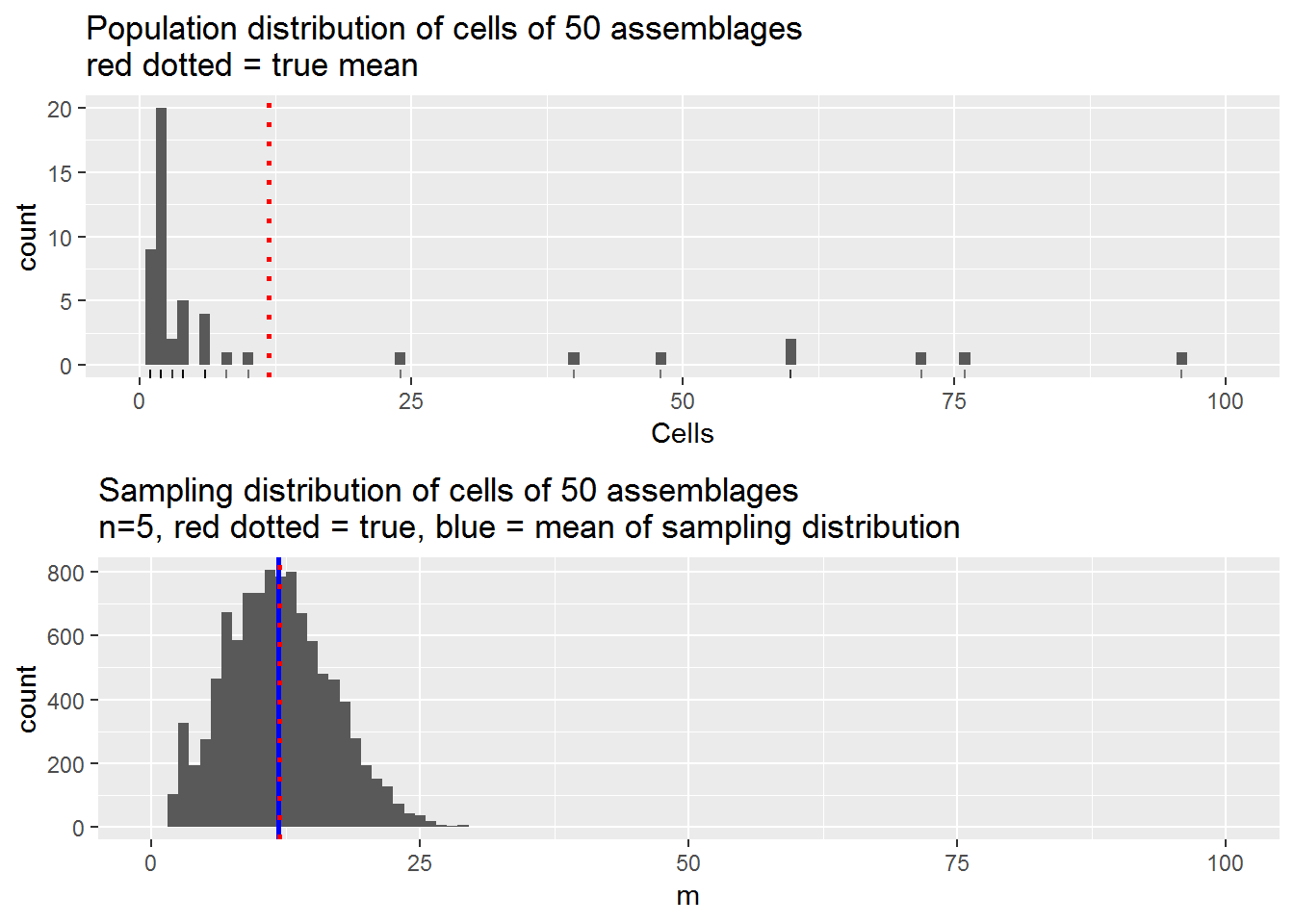# Earth’s water example

## Sample from a sphere

First, we will discuss methods of sampling from points on the beach ball in order to determine the proportion of water (blue regions) on the surface.

In class we sampled the beach ball and observed $$x = ?$$ of $$n = ?$$ observations were water.

## notes for prop.test() and binom.test()
# x = number of "successes"
# n = total sample size
n = 2
x = 1

dat.globe <- data.frame(type = c("Water", "Land"), freq = c(x, n - x), prop = c(x, n - x) / n)
dat.globe
##    type freq prop
## 1 Water    1  0.5
## 2  Land    1  0.5
# binom.test() is an exact test for a binomial random variable
b.summary <- binom.test(x = x, n = n, p = 0.5, conf.level = 0.95)
b.summary
##
##  Exact binomial test
##
## data:  x and n
## number of successes = 1, number of trials = 2, p-value = 1
## alternative hypothesis: true probability of success is not equal to 0.5
## 95 percent confidence interval:
##  0.01257912 0.98742088
## sample estimates:
## probability of success
##                    0.5
library(ggplot2)
p <- ggplot(data = subset(dat.globe, type == "Water"), aes(x = type, y = prop))
p <- p + geom_hline(yintercept = c(0, 1), alpha = 1/4)
p <- p + geom_bar(stat = "identity")
p <- p + geom_errorbar(aes(min = b.summary$conf.int, max = b.summary$conf.int), width=0.25)
p <- p + geom_hline(yintercept = 0.71, colour = "red")
p <- p + scale_y_continuous(limits = c(0, 1))
p <- p + coord_flip() # flip the x and y axes for horizontal plot
print(p)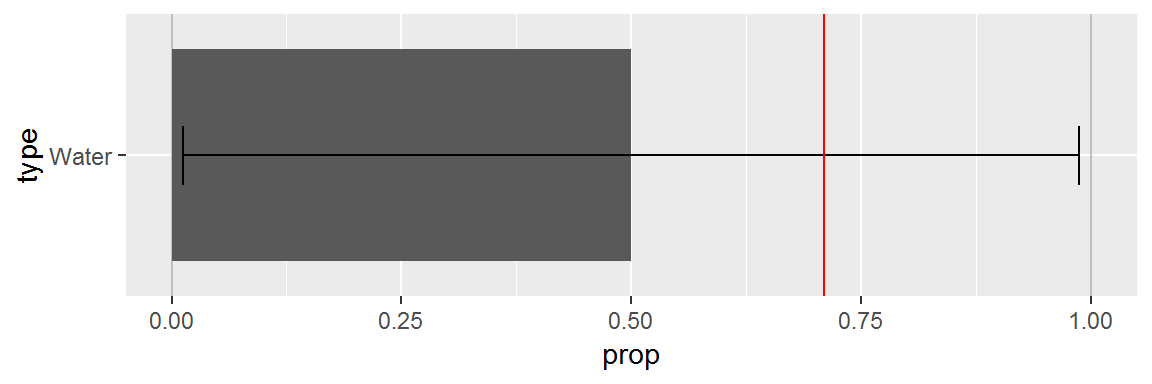## Questions to answer

1. (2 p) Informally, determine whether the sampling strategy led to an unbiased or a biased estimate of the true proportion of water on the beach ball (assuming the ball has the same as the true amount of water on the earth’s surface, 71%).

In later weeks we will learn how to conduct a confidence interval to determine whether the sample was consistent with the population.

# African countries in the UN example

Previously in class we collected data using a randomized experiment. We provided a priming number (X = 10 or 65, not actually a random number) then asked you two questions:

1. Do you think the percentage of countries represented in the United Nations that are from Africa is higher or lower than X?

2. Give your best estimate of the percentage of countries represented in the United Nations that are from Africa.

The data were compiled into either the text table below or a csv file on the website and we read it directly.

# dat.UN.Africa <-
#   read.table(text = "
# PrimingNumber HighLow UN_Percentage
# 10  L 25
# 10  H 12
# 10  H 7
# 65  L 25
# 65  H 12
# 65  H 7
# ", header = TRUE)

dat.UN.Africa <-
read_csv("https://statacumen.com/teach/S4R/worksheet/S4R_WS_16_Sampling_UN_Experiment_data.csv")
## Parsed with column specification:
## cols(
##   PrimingNumber = col_double(),
##   HighLow = col_character(),
##   UN_Percentage = col_double()
## )
dat.UN.Africa$PrimingNumber <- factor(dat.UN.Africa$PrimingNumber)
dat.UN.Africa$HighLow <- factor(dat.UN.Africa$HighLow, levels = c("L", "H"))

str(dat.UN.Africa)
## Classes 'spec_tbl_df', 'tbl_df', 'tbl' and 'data.frame': 19 obs. of  3 variables:
##  $PrimingNumber: Factor w/ 2 levels "10","65": 2 1 2 1 1 1 2 2 2 1 ... ##$ HighLow      : Factor w/ 2 levels "L","H": 1 2 1 2 2 2 1 1 1 1 ...
##  \$ UN_Percentage: num  12 70 35 50 15 30 5 10 25 8 ...
##  - attr(*, "spec")=
##   .. cols(
##   ..   PrimingNumber = col_double(),
##   ..   HighLow = col_character(),
##   ..   UN_Percentage = col_double()
##   .. )

Here are some summaries and plots.

## If we create a summary data.frame with a similar structure as our data, then we
##   can annotate our plot with those summaries.
# calculate the estimated mean and order
mean.UN.Africa <-
dat.UN.Africa %>%
group_by(PrimingNumber) %>%
summarize(UN_Percentage = mean(UN_Percentage)) %>%
ungroup()
mean.UN.Africa
## # A tibble: 2 x 2
##   PrimingNumber UN_Percentage
##   <fct>                 <dbl>
## 1 10                     28.8
## 2 65                     19
# histogram using ggplot
library(ggplot2)
p <- ggplot(dat.UN.Africa, aes(x = UN_Percentage))
p <- p + geom_histogram(binwidth = 4)
p <- p + geom_rug()
p <- p + geom_vline(data = mean.UN.Africa, aes(xintercept = UN_Percentage), colour = "red")
p <- p + facet_grid(PrimingNumber ~ .)
print(p)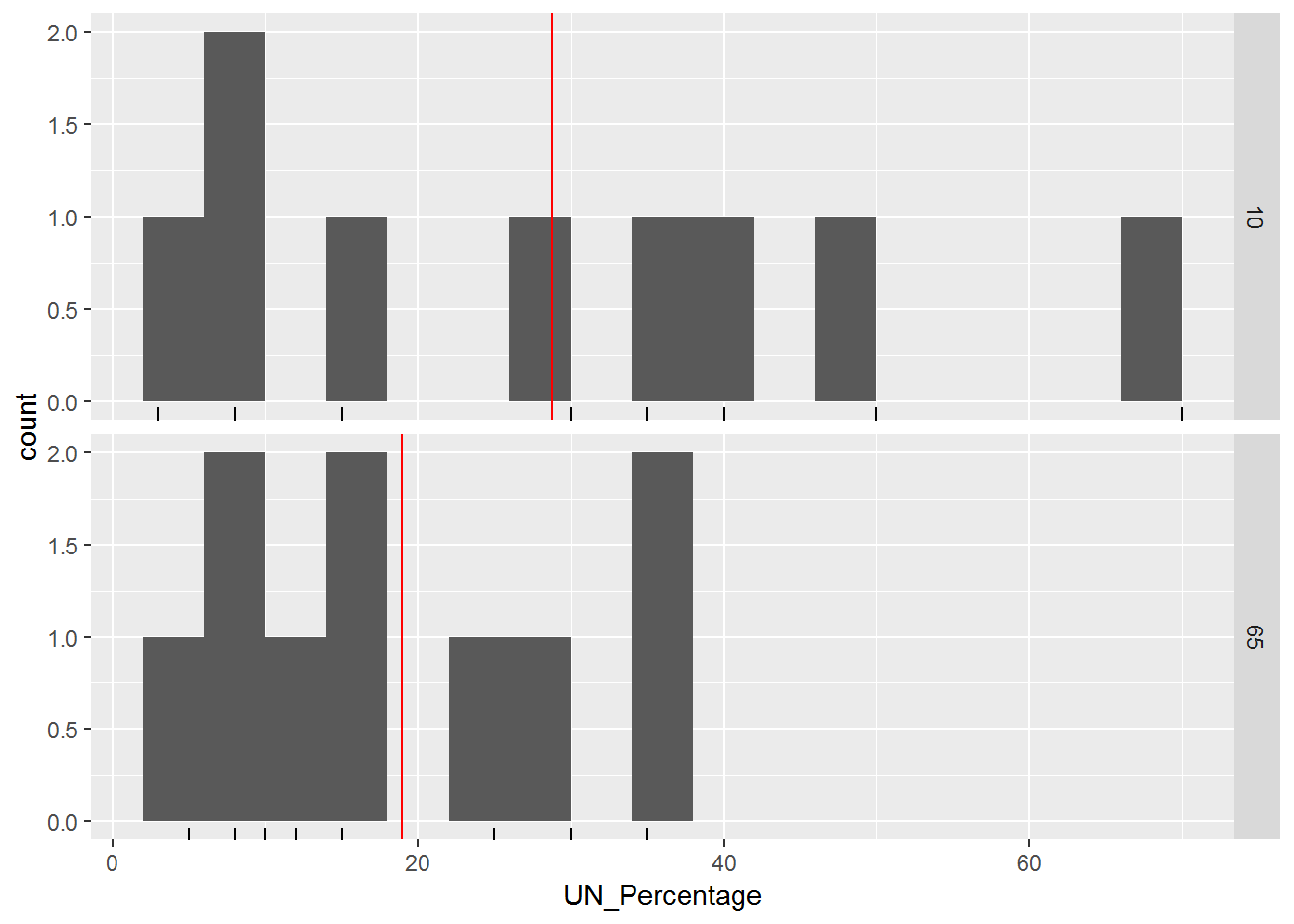# p <- ggplot(dat.UN.Africa, aes(x = UN_Percentage, fill=PrimingNumber))
# p <- p + geom_histogram(binwidth = 4, alpha = 0.5, position="identity")
# p <- p + geom_rug()
# p <- p + geom_vline(data = mean.UN.Africa, aes(xintercept = UN_Percentage, colour = PrimingNumber, linetype = PrimingNumber))
# p <- p + geom_rug(aes(colour = PrimingNumber), alpha = 1/2)
# print(p)

## Questions to answer

A priori, before we observed the data, we hypothesized that those who were primed with a larger number (65) would provide a higher percentage (UN_Percentage) than those with the lower number (10). Therefore, this is a one-sided test.

1. (2 p) Is there a suggestion that priming had an effect on the responses?

In later weeks we will learn how to conduct a formal hypothesis test to make this decision.